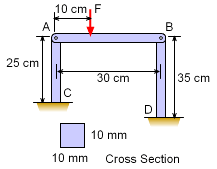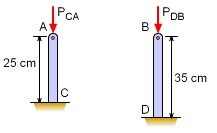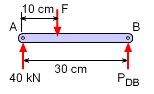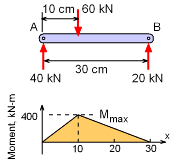Ch 9. Columns Multimedia Engineering Mechanics BasicColumns FixedColumns EccentricLoads
 Chapter 1. Stress/Strain 2. Torsion 3. Beam Shr/Moment 4. Beam Stresses 5. Beam Deflections 6. Beam-Advanced 7. Stress Analysis 8. Strain Analysis 9. Columns Appendix Basic Math Units Basic Equations Sections Material Properties Structural Shapes Beam Equations Search eBooks Dynamics Fluids Math Mechanics Statics Thermodynamics Author(s): Kurt Gramoll ©Kurt GramollMECHANICS - EXAMPLESupport Structure Example A mechanical equipment support structure is made from three metal members, each 10 mm by 10 mm. The vertical members, CA and DB, are fixed to the ground. The horizontal member, AB, is pinned to the vertical members at A and B. If the equipment is expected to placed 10 cm from the the left, as shown, what is the maximum load F? Assume all columns are solid steel bars with a stiffness, E, of 200 GPa and a allowable stress of 400 MPa. SolutionBasic W8x31 I-Beam With the slender vertical columns, 10 mm by 10 mm, it would be expect this structure to fail due to column buckling. However, it could also fail due to beam bending or column crushing. These other possible failure modes will be checked after load P is determined for just column buckling. Both columns, CA and DB, can be considered as fixed-pinned columns that have a critical buckling as      Pcr = 2.046 π2EI / L2 Moment of inertia for both columns is,      I = ab3 / 12 = (0.01)(0.01)3 / 12 = 8.333x10-10 m4 The allowable buckling load for each column is       PCA = 2.046 π2 (200x109) (8.333x10-10)/0.252              = 53.85 kN      PBD = 2.046 π2 (200x109) (8.333x10-10)/0.352             = 27.47 kNTop Beam Section Now, the critical buckling loads can be used to find F. First, the PCA will be used to find F, and then PBD. Taking moments about A gives,       (10)F = PBD(30) = 27.47(30)       P = 82.41 kN Next, using PBD and taking moments about B gives,        (20)F = PAC(30) = 53.85(30)        F = 80.78 kN Thus the largest load F before either column will be buckle when F = 80.78 kN.Maximum Crushing Load, 40 kN, in Column CA Check column normal stress (crushing stress):      σ = P/A = 80.78/0.012 = 807 MPa There is a problem. This is higher than the allowable of 400 MPa. It will fail due to crushing before buckling. The maximum column load is,       P = (400 MPa) (0.01 m) (0.01 m) = 40 kN This means the largest F (moments about point B) will be      20(F) = 40(30)      F = 60 kNMaximum Bending Moment Check beam bending stress: The largest bending moment due to a load of F = 60 kN (maximum due to column crushing) will be at the location of the load.       Mmax = 40(10) = 400 kN-m The bending stress will be      σ = My/I         = (400 kN-m) (0.005 m) / 8.333x10-10 m4         = 2,400,000 MPa There is another serious problem. This is much higher than the allowable of 400 MPa. It will fail due to beam bending before buckling or column crushing. The maximum moment is,      400 MPa = M (0.005 m) / 8.333x10-10 m4      M = 66.66 N-m This moment will be generated a reaction at A of      PA = (66.66 N-m)/ (0.1 m) = 666.66 N Thus, the maximum load F will be      F (20) = 666.6 (30)      F = 999.9 N = 1.0 kN Thus, the structure will fail due to beam bending before column buckling or column crushing. It is important to check all failure modes.

Practice Homework and Test problems now available in the 'Eng Mechanics' mobile app
Includes over 400 problems with complete detailed solutions.
Available now at the Google Play Store and Apple App Store.